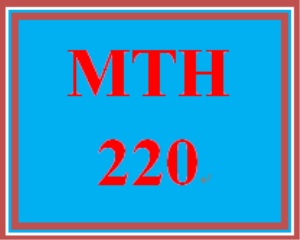# MTH 220T Wk 5 – Final Exam

PLDZ-12932 Free In Stock
\$ 0.00 USD
Description

***************************************************************

https://uopcourse.com/category/mth-220/  ~~~~~~~~~~~~~~~~~~~~~~~~~~~~~~~~~~~~~~~~~~~~~~~~~

https://uopcourse.com/

***************************************************************

MTH 220T Wk 5 – Final Exam

Complete the final exam. You have one attempt at the exam. So, be sure to review all previous course materials before attempting the exam.

Question 1

10 Points

How many four-character passwords can be formed using the characters A, B, C, 1, 2 if the characters can be repeated?

256

625

120

1024

Question 2

10 Points

Find the radius of the circle 5 x squared plus 5 y squared plus 10 x minus 20 y equals 55 .

r equals 16

r equals 4

r equals 35

r equals 6

Question 3

10 Points

Find the sum of the first six terms in the sequence {1, 5, 9, 13, ...}.

66

21

60

11

Question 4

10 Points

Find the sum of the following infinite series:

begin inline style sum from i equals 1 to infinity of end style 5 times 0.2 to the power of i minus 1 end exponent

25

5

Question 5

10 Points

Find the equation of a horizontal parabola that has vertex (-3, -4) and distance to the focus p equals 1 half .

x equals 1 half left parenthesis y minus 3 right parenthesis squared plus 4

x equals negative 1 half left parenthesis y minus 4 right parenthesis squared minus 3

x equals 1 half left parenthesis y plus 4 right parenthesis squared minus 3

x equals negative 1 half left parenthesis y plus 3 right parenthesis squared minus 4

Question 6

10 Points

Find the sum of the following series:

begin inline style sum from n equals 1 to 30 of end style 2 n

435

900

465

930

Question 7

10 Points

Find the sum of the first five terms using the geometric series formula for the sequence left curly bracket 1 half comma space minus 1 comma 2 comma negative 4 comma... right curly bracket .

11 over 2

11 over 32

fraction numerator negative 31 over denominator 6 end fraction

33 over 2

Question 8

10 Points

Convert the equation 6 x squared minus 3 y squared plus 12 x minus 18 y minus 3 equals 0 to the standard form of a hyperbola.

fraction numerator 2 left parenthesis x plus 1 right parenthesis squared over denominator 1 end fraction plus fraction numerator left parenthesis y plus 3 right parenthesis squared over denominator 1 end fraction equals 1

fraction numerator left parenthesis x minus 1 right parenthesis squared over denominator 3 end fraction minus fraction numerator left parenthesis y minus 3 right parenthesis squared over denominator 6 end fraction equals 1

fraction numerator left parenthesis y plus 3 right parenthesis squared over denominator 6 end fraction minus fraction numerator left parenthesis x plus 1 right parenthesis squared over denominator 3 end fraction equals 1

fraction numerator 3 left parenthesis y plus 3 right parenthesis squared over denominator 13 end fraction minus fraction numerator 6 left parenthesis x plus 1 right parenthesis squared over denominator 13 end fraction equals 1

Question 9

10 Points

Find the equations of the asymptotes of the hyperbola x squared over 25 minus y squared over 16 equals 1 .

y equals 4 over 5 x space a n d space y equals negative 4 over 5 x

y equals 5 over 4 x space a n d space y equals negative 5 over 4 x

y equals 5 over 4 x minus 1 space a n d space y equals negative 5 over 4 x minus 1

y equals 4 over 5 x plus 1 space a n d space y equals negative 4 over 5 x plus 1

Question 10

10 Points

Evaluate begin inline style sum from n equals 3 to 7 of end style left parenthesis n squared plus 2 n right parenthesis .

Recent Reviews Write a Review
0 0 0 0 reviews